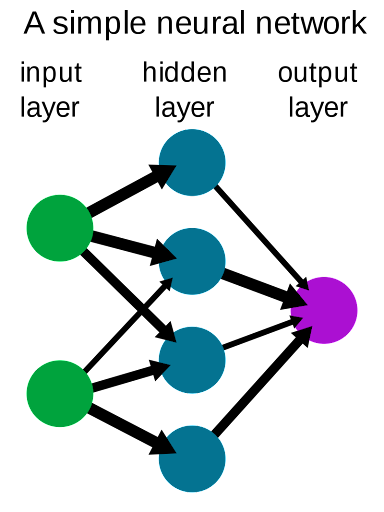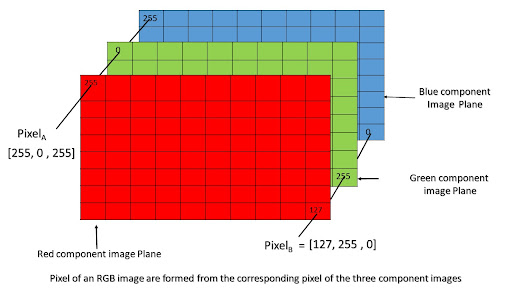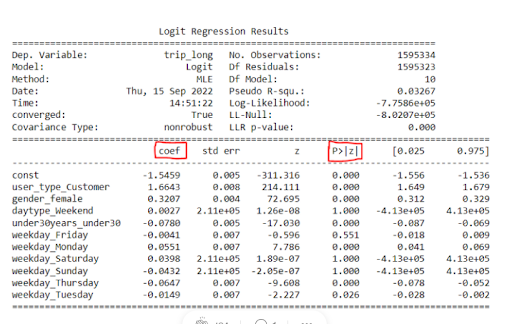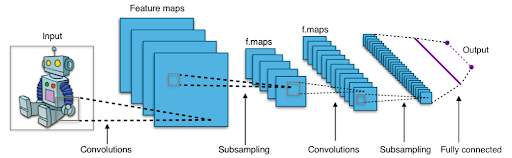# Deep Learning Interview Questions

## Overview

Deep learning is typically used as a marketing term, diluting its original meaning and creating a cognitive dissonance within its concepts.

Nevertheless, it serves as a foothold within current technologies, powering many tools we take for granted—matching algorithms in dating apps, predictive models in Youtube and TikTok’s For You page, and pricing algorithms in Uber’s surge pricing calculations are only a few examples of AI’s dominance in the current market.

It is not surprising that the demand for deep learning–related jobs has spiked over the years as these models improve company profits and customer retention. This article will help you prepare for your next deep-learning job interview, covering the basics and beyond.

## What Is Deep Learning and How Does It Work?

Deep learning neural networks are intricate structures with nodes called neurons made to simulate the human brain’s neural system. At their core, the neurons in these complex networks are a mathematical function that modifies a value based on a specific mathematical structure.

A layer in a neural network is a group of neurons that operates together at a certain depth within an artificial neural network (ANN). Typical ANNs have a depth of three layers (including the input and output layers). However, any ANN that extends from a depth of three layers is called a deep learning neural network.

At its core, a deep-learning neural network is a cluster of nodes with vast nested abstract layers that take input data and generate an output using mathematical models for function approximation.

## Why Is Deep Learning Important?

Deep learning neural networks are incredibly flexible, and for most people, they are the go-to solution to any machine learning (ML) problem, although this isn’t recommended.

In a sense, deep learning is fundamental because it allows us to develop technologies that transcend from hard-coded nested if…else and switch…case statements.

For example, if you were to build a natural language processing (NLP) algorithm without ML that compresses a line of text to include only the substantial words and use it to generate some sentiment analysis, it would look like the following:

``````//pseudo-algorithm
std::string sentence[word_count]; // contains the input sentence
int sentiment = 0;
std::string compressed_sentence[word_count]; // will hold the output
int index = 0;
std::string english_syntactic_sugar[size]; // contains unsubstantial words in the English language
for (int i=0; i<word_count; i++){
if (sentence[i]=="good"){
sentiment += 5;
compressed_sentence[index] = sentence[i];
index++;
sentiment -= 5;
compressed_sentence[index] = sentence[i];
index++;
} else if {
bool useless_word = false;
for (int j=0; j<size; j++){
if (english_syntactic_sugar[j]==sentence[i]){
useless_word = true;
break;
}
if (useless_word){
continue;
}
}
} else if ... // more else ifs checking a word and manually assigning its sentiment value
}
``````

However, this algorithm has a critical flaw: it can inaccurately assign sentiment values if the context differs. For example, a comment stating, “This product is NOT good,” would be assigned a positive sentiment value because it has the word good in it. This is incorrect, though, the comment should be assigned a negative sentiment value.

To avoid this logical error, you will need to hard code another if…else statement that checks whether the word “not” was placed before the word “good.”

To alleviate this coding spiral, deep learning can help streamline this process and learn to identify the patterns wherein a sentence structure has a positive sentiment value or a negative sentiment value.

Deep learning neural networks can help train networks to do something instead of needing specific manually-coded algorithms. This is especially important when there are a lot of cases and variables to consider, wherein manually coding each case is virtually impossible.

## What Are the Different Types of Neural Networks in Deep Learning?

Many neural networks are designed to take advantage of the added efficiency and accuracy of deep learning and its inherent ability to identify features. The three most often used neural networks are:

• Convolutional neural networks (CNNs): Specialize in images and computer vision utilizes convolution layers
• Multilayer perceptrons: Feed-forward and have the same number of input and output nodes
• Long short-term memory networks: Can retain and recall information over long periods

## Basic Deep Learning Interview Questions

### 1. What are ANNs?Artificial neural networks are models that simulate how the human brain functions and operates. Typically, ANNs contain a few hundred to a few million nodes, depending on the number of features to identify and train.

ANNs are first structured with the input layer (that takes input from training datasets), which is then processed by the hidden layers. Hidden layers are the bread and butter of deep learning neural networks and perform forward and backward passes to limit the margin of error.

The output layer outputs a result of a probability and holds its weights and biases before the final output is provided.

### 2. When Should Deep Learning Neural Networks Not Be Used?

Because deep learning neural networks are so flexible, people frequently try to use them for anything related to AI. Although it is true that utilizing deep learning often results in better-quality models, there are certain conditions wherein it can be counterintuitive.

• Deep learning with smaller models is typically less efficient than simpler machine learning models. Although not necessarily worse, it requires more processing power for a slight improvement.
• Because of the nested hidden layers, deep learning models require more training time, and as such may not be helpful if training time is limited.
• Deep learning models require GPUs to function appropriately, and training might not be so terse without the proper hardware.

### 3. What are the Differences Between Machine Learning and Neural Networks?

Machine learning and deep learning are not inherently different; they aren’t even separate entities. Deep learning is a subset of machine learning, not a separate field, and comparing them may not be logically correct. As such, for the rest of this article, any terms related to machine learning refer to non-deep learning machine learning models.

• Compared to deep learning, machine learning can function better with lower-spec hardware.
• Machine learning also is typically cheaper to run and develop. However, this may not be of concern when utilizing pre-built libraries (i.e., Tensorflow).
• Deep learning utilizes many layers and heavy GPU processing to provide more accurate results than machine learning.
• Deep learning can perform unsupervised learning to develop and discover patterns. However, this may result in heightened requirements for training datasets

## Intermediate Deep Learning Interview Questions

### 1. What Are the Types of Tensors?

There are three types of tensors:

• Variables are tensors that are designed to change throughout the runtime of your algorithm/program. Typically, these are the trainable parameters of your model.
• Constants are tensors that remain the same throughout the operation of a program. While it may seem counterintuitive to limit your control over your tensors by declaring them constants, a general rule for computing is always to use the least powerful option possible. By doing so, you are ensuring that data that are not immutable by nature (i.e., mathematical constants, formulas) are not replaced later down the line.
• Placeholders are tensors with no inherent value at the start but are declared as a promise that these tensors will soon be filled with relevant data.

### 2. What Is the Difference Between Feed-Forward Neural Networks and Recurrent Neural Networks?

A feed-forward neural network is a neural network wherein data flow from the input node to the output node, and the data flow is not circular (i.e., the connections between the nodes do not form a cycle).

This type of neural network is more straightforward than recurrent neural networks and only considers the current input to generate the output. This approach is unlike recurrent neural networks, wherein previous inputs and outputs are considered in calculating the current output.

On the other hand, recurrent neural networks can loop through inputs and outputs because the neurons are looped together. These networks are dynamic as their states continuously change until they reach some stasis. Until a new input is fed, it will remain at this equilibrium point.

Typically, feed-forward neural networks are used for pattern recognition, whereas recurrent neural networks are better suited for determining how the number of inputs changes or shifts the output.

## Advanced Deep Learning Interview Questions

### 1. Airbnb: Brazilian Bookings Recommendations (From Interview Query’s Takehomes)Background:

Knowing which home listings to recommend to a guest could provide huge business value to Airbnb. Therefore, ​we would like to train a recommender model that can predict which listings a specific user is likely to book​. The dataset provided here contains a random sample of our 7-day search log from two markets: Rio de Janeiro and São Paulo

Every time a user conducts a search they are shown a certain number of listings that are available for the searched location, dates and search filters.

Given the search results, the user can conduct one or more actions on a specific listing: impress (no action), click (which takes the user to the listing page), contact host (to inquire about the listing), and finally, book.

### 2. How Is Data Structured and Represented in Deep Learning?

Data are typically represented as tensors in deep learning. Tensors are a general term for n-dimensional arrays of data, and they can be your standard vector (one-dimensional), matrix (two-dimensional), or cuboid (three-dimensional).

Despite the complex dimensions, these input data are typically processed as a continuous piece of data with multiple indexes for more straightforward iteration and access. So, technically, a matrix can be represented as a vector. However, whether the inputs are processed as a continuous piece of data depends on the implementation; modern, high-level implementations of tensors and other n-dimensional data structures are not contiguous.

### 3. How Do CNNs Process Images?

CNNs do not process images as-is because images on their own, when viewed as a visual object, cannot have computable values that a neural network can use. However, images have inherent numeric values using the RGB scale (i.e., red, green, and blue).

Most images in your dataset will undoubtedly be 8-bit (although commercial 16-bit photos are becoming more common). A pixel’s color can be determined by how strong the red, green, and blue hues are. Most colors can be represented with just red, green, and blue; orange, for example, has a code of RGB(255,165,0).

This image is then represented in three two-dimensional matrices, one matrix for red, another for green, and another for blue. However, CNNs still cannot take this as-is; this image is too detailed and heavy for CNNs to calculate.A modern iPhone camera has 12 megapixels, which means it has 12 million pixels, and each pixel will hold three color values (RGB). Each color value will be one byte each, which can be calculated as 36 megabits per picture.

The problem is that hundreds to thousands of pictures are needed to train a model. Therefore, training a CNN with raw images is unfeasible. That is why convolutions are used, to help reduce the size of these images while retaining vital information to help generate a simplified, flattened matrix for the CNN to work while maintaining accuracy.

### 4. When Designing Neural Networks for Optimization, How Does the Adam Optimizer Differ From Other Optimization Methods? (From Interview Query)

It is also important to consider what the benefits are of using the Adam optimization algorithm as opposed to other optimization methods.

Adam optimizer combines SDG with momentum and RMSprop. After each iteration, the gradient is computed using the RMSprop exponential moving average of the gradient in the numerator, and the square root (plus a small number to avoid dividing by zero) of the RMSprop computed gradient. RMSprop gradient is similar to SDG with momentum except that we add the square of the gradient to the momentum term.

### 5. How Would You Interpret Coefficients of Logistic Regression for Categorical and Boolean Variables? (From Interview Query)

Coefficient means log odds ratio = log(probability of occuring/1-prob)

For categorical features or predictors, the odds ratio compares the odds of the event occurring for each category of the predictor relative to the reference category, given that all other variables remain constant.### 6. What Is the Architecture Behind CNNs?

Convolutional neural networks have layers to process images, which helps them determine the patterns without explicitly labeling images. They deal with the following layers in their architecture:CNNs have the input layer to gather the image’s information. In contrast, images may be associated with something visual; these networks process images mathematically. Refer to the “How do CNNs process images?” question. The mathematical data are transferred from the input layer to the convolution layer.

The convolution layer merges two features (i.e., red and blue values in an image) to create a feature map. A CNN can have multiple convolutions. These convolutions also have the added benefit of compression.

The pooling layer is in charge of reducing dimensionality and the number of parameters. This is beneficial because it reduces the resources needed for training.

The fully connected layers receive the flattened tensor (after all those convulsions), start performing the mathematical processes, and then add a Softmax function at the output.